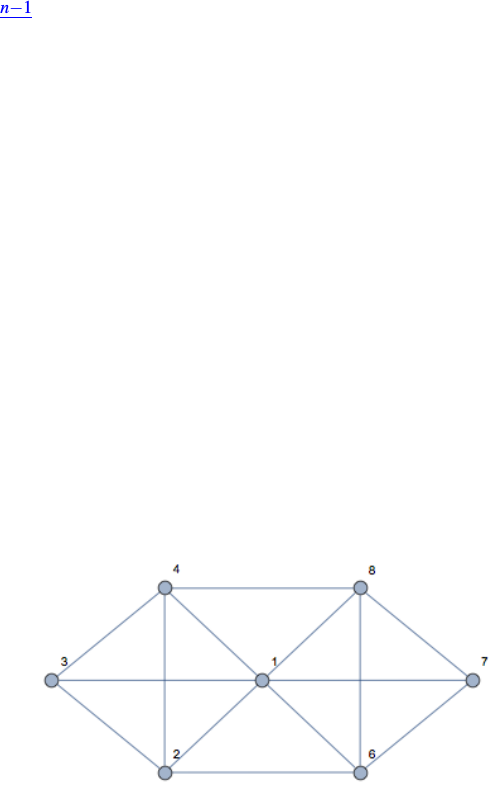Get 2 days of premium access
Study Guides (380,000)
US (220,000)
Berkeley (5,000)
COMPSCI (500)
Quiz

# COMPSCI 70 Study Guide - Quiz Guide: Alistair Sinclair, Complete Graph, Disjoint SetsExam

Department
Computer Science
Course Code
COMPSCI 70
Professor
Rao Satish
Study Guide
Quiz

This preview shows pages 1-2. to view the full 6 pages of the document.CS 70 Discrete Mathematics and Probability Theory
Fall 2018 Alistair Sinclair and Yun Song HW 3
1 Short Answer: Graphs
(a) Bob removed a degree 3 node in an n-vertex tree, how many connected components are in the
resulting graph? (An expression that may contain n.)
(b) Given an n-vertex tree, Bob added 10 edges to it, then Alice removed 5 edges and the resulting
graph has 3 connected components. How many edges must be removed to remove all cycles
in the resulting graph? (An expression that may contain n.)
(c) True or False: For all n3, the complete graph on nvertices, Knhas more edges than the
n-dimensional hypercube. Justify your answer.
(d) A complete graph with nvertices where nis an odd prime can have all its edges covered with
xedge-disjoint Hamiltonian cycles (a Hamiltonian cycle is a cycle where each vertex appears
exactly once). What is the number, x, of such cycles required to cover the a complete graph?
(Answer should be an expression that depends on n.)
(e) Give a set of edge-disjoint Hamiltonian cycles that covers the edges of K5, the complete graph
on 5 vertices. (Each path should be a sequence (or list) of edges in K5, where an edge is written
as a pair of vertices from the set {0,1,2,3,4}- e.g: (0,1),(1,2).)
Solution:
(a) 3.
Each neighbor must be in a different connected component. This follows from a tree having a
unique path between each neighbor in the tree as it is acyclic. The removed vertex broke that
path, so each neighbor is in a separate component. Moreover, every other node is connected
to one of the neighbors as every other vertex has a path to the removed node which must go
through a neighbor.
(b) 7
The problem is asking you to make each component into a tree. The components should have
n11, n21 and n31 edges each or a total of n3 edges. The total number of edges after
Bob and Alice did their work was n1+10 5=n+4, thus one needs to remove 7 edges to
ensure there are no cycles.
(c) False
This is just an exercise in deﬁnitions. The complete graph has n(n1)/2 edges where the
hypercube has n2n1edges. For n3, 2n1(n1)/2.
CS 70, Fall 2018, HW 3 1

Only pages 1-2 are available for preview. Some parts have been intentionally blurred.(d) (n1)/2.
Each cycle removes degree 2 from each vertex. As the degree of each vertex is n1, we
require a total of n1
2disjoint cycles. This is also sufﬁcient. For a construction in the case of n
being an odd prime, see explanations below.
(e) (0,1),(1,2),(2,3),(3,4),(4,0)
(0,2),(2,4),(4,1),(1,3),(3,0)
The following details a procedure for generating the paths using ideas from modular arithmetic.
Note that modular arithmetic is not necessary for the solution, but it provides a clean solution.
The idea is that we can generate disjoint Hamiltonian cycles by repeatedly adding an element a
to the current node. This produces the sequence of edges (0,a),(a,2a),...,((p1)a,0)which
are disjoint for different a, as long as a6=a(mod p), as that would simply be subtracting a
everytime. (In other words, there exists no integer ksuch that a+pk =a.)
We use primality to say that inside a sequence the edges are disjoint since the elements
{0a,...,(p1)a}are distinct (mod p).
2 Eulerian Tour and Eulerian Walk
(a) Is there an Eulerian tour in the graph above?
(b) Is there an Eulerian walk in the graph above?
(c) What is the condition that there is an Eulerian walk in an undirected graph? Brieﬂy justfy your
answer.
Solution:
(a) No. Two vertices have odd degree.
(b) Yes. One of the two vertices with odd degree must be the starting vertex, and the other one
must be the ending vertex. For example: 3,4,2,1,3,2,6,1,4,8,1,7,8,6,7 will be an Eulerian
walk (the numbers are the vertices visited in order). Note that there are 14 edges in the graph.
CS 70, Fall 2018, HW 3 2
###### You're Reading a Preview

Unlock to view full version